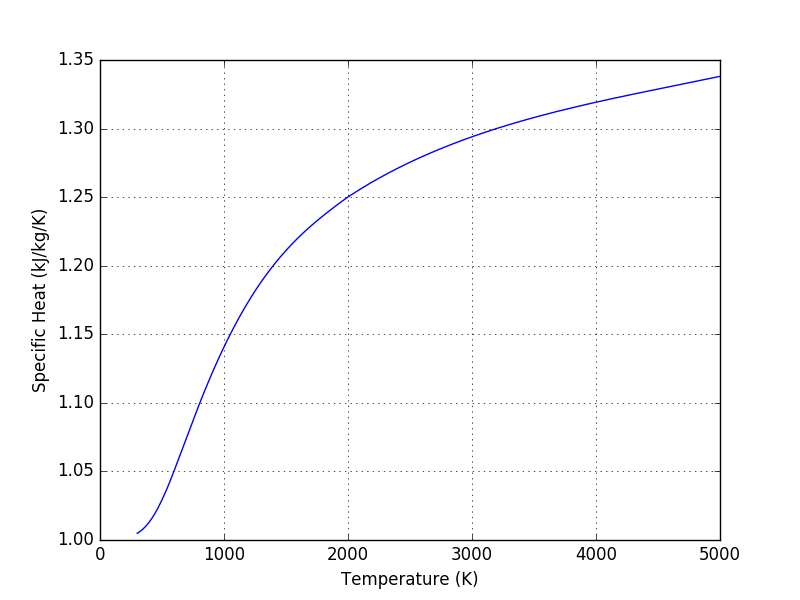# PYroMat## Thermodynamic Properties in Python

Simple, accurate, and versatile
thermodynamic properties
in Python
```>>> import pyromat as pm
>>> H2O = pm.get('mp.H2O')
>>> h = H2O.h(T=450., p=3.)```
Access any of
934 substances
in only a few lines of Python
PYroMat's array support
makes plotting
and working with big data sets
easy.
```>>> import numpy as np
>>> O2 = pm.get('ig.O2')
>>> import numpy as np
>>> T = np.linspace(300.,2000.,101)
>>> h = O2.h(T)
>>> h.shape
(101,)
>>> p = np.linspace(1.,100.,101)
>>> s = O2.s(T,p)
>>> s.shape
(101,)
>>> T = T.reshape((T.size,1))
>>> s = O2.s(T,p)
>>> s.shape
(101, 101)```
```>>> import matplotlib.pyplot as plt
>>> T = np.linspace(300.,5000.,201)
>>> air = pm.get('ig.air')
>>> plt.plot(T, air.cp(T))```